# Excel XIRR function to find internal rate of return for non-periodic cash flows

The tutorial shows how to use XIRR in Excel to calculate the internal rate of return (IRR) for cash flows with irregular timing and how to make your own XIRR calculator.

When you are faced with a capital-intensive decision, calculating the internal rate of return is desirable because it lets you compare the projected returns for different investments and gives a quantitative basis for making the decision.

In our previous tutorial, we looked at how to calculate the internal rate of return with the Excel IRR function. That method is quick and straightforward, but it has an essential limitation – the IRR function assumes that all cash flows occur at equal time intervals such as monthly or annually. In real life situations, however, cash inflows and outflows often happen at irregular intervals. Thankfully, Microsoft Excel has another function to find IRR in such cases, and this tutorial will teach you how to use it.

## XIRR function in Excel

The Excel XIRR function returns the internal rate of return for a series of cash flows that may or may not be periodic.

The function was introduced in Excel 2007 and is available in all later versions of Excel 2010, Excel 2013, Excel 2016, Excel 2019, and Excel for Office 365.

The syntax of the XIRR function is as follows:

XIRR(values, dates, [guess])

Where:

• Values (required) – an array or a range of cells that represent a series of inflows and outflows.
• Dates (required) – dates corresponding to cash flows. Dates may occur in any order, but the date of the initial investment must be first in the array.
• Guess (optional) – an expected IRR supplied as a percentage or decimal number. If omitted, Excel uses the default rate of 0.1 (10%).

For example, to calculate IRR for the series of cash flows in A2:A5 and dates in B2:B5, you'd use this formula:

`=XIRR(A2:A5, B2:B5)`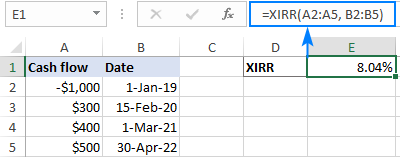Tip. For the result to display correctly, please make sure the Percentage format is set for the formula cell.

### 6 things you should know about XIRR function

The following notes will help you better understand the inner mechanics of the XIRR function and use it in your worksheets most efficiently.

1. XIRR in Excel is designed for calculating the internal rate of return for cash flows with unequal timing. For periodic cash flows with exact payment dates unknown, you can use the IRR function.
2. The range of values must contain at least one positive (income) and one negative (outgoing payment) value.
3. If the first value is an outlay (initial investment), it must be represented by a negative number. The initial investment is not discounted; subsequent payments are brought back to the date of the first cash flow and discounted based on a 365-day year.
4. All dates are truncated to integers, meaning that the fractional part of a date that represents time is removed.
5. Dates must be valid Excel dates entered as references to cells containing dates or results of formulas such as the DATE function. If dates are input in the text format, problems may occur.
6. XIRR in Excel always returns an annualized IRR even when calculating monthly or weekly cash flows.

## XIRR calculation in Excel

The XIRR function in Excel uses a trial and error approach to find the rate that satisfies this equation: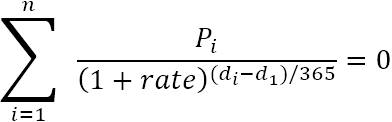Where:

• P - cash flow (payment)
• d - date
• i - period number
• n - periods total

Starting with the guess if provided or with the default 10% if not, Excel goes through iterations to arrive at the result with 0.000001% accuracy. If after 100 attempts an accurate rate is not found, the #NUM! error is returned.

To check the validity of this equation, let's test it against the result of the XIRR formula. To simplify our calculation, we will be using the following array formula (please remember that any array formula must be completed by pressing Ctrl + Shift + Enter):

`=SUM(A2:A5/((1+\$E\$1)^((B2:B5-\$B\$2)/365)))`

Where:

• A2:A5 are the cash flows
• B2:B5 are the dates
• E1 is the rate returned by XIRR

As shown in the screenshot below, the result is very close to zero. Q.E.D. :)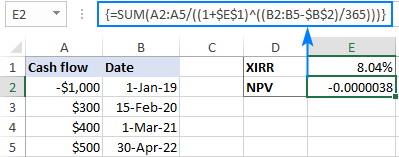## How to calculate XIRR in Excel – formula examples

Below are a few examples that demonstrate the common uses of the XIRR function in Excel.

### Basic XIRR formula in Excel

Suppose you invested \$1,000 in 2017 and expect to receive some profit in the next 6 years. To find the internal rate of return for this investment, use this formula:

`=XIRR(A2:A8, B2:B8)`

Where A2:A8 are cash flows and B2:B8 are the dates corresponding to the cash flows: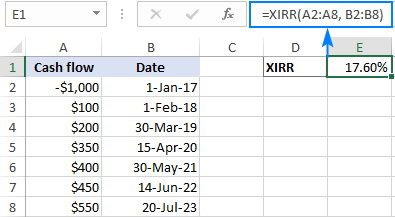To judge the profitability of this investment, compare the XIRR output with your company's weighted average cost of capital or hurdle rate. If the returned rate is higher than the cost of capital, the project can be considered a good investment.

When comparing several investment options, please remember that a projected return rate is just one of the factors that you should estimate before making a decision. For more information, please see What is the internal rate of return (IRR)?

### Complete form of Excel XIRR function

In case you know what kind of return you are expecting from this or that investment, you can use your expectation as a guess. It is especially helpful when an obviously correct XIRR formula throws a #NUM! error.

For the data input shown below, an XIRR formula without the guess returns an error:

`=XIRR(A2:A7, B2:B7)`

The anticipated return rate (-20%) put in the guess argument helps Excel arrive at the result:

`=XIRR(A2:A7, B2:B7, -20%)`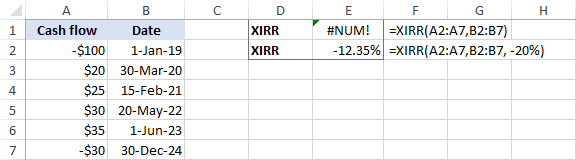### How to calculate XIRR for monthly cash flows

For starters, please remember this – whatever cash flows you are calculating, the Excel XIRR function produces an annual rate of return.

To make sure of this, let's find IRR for the same series of cash flows (A2:A8) that occur monthly and yearly (the dates are in B2:B8):

`=XIRR(A2:A8, B2:B8)`

As you can see in the below screenshot, the IRR goes from 7.68% in case of annual cash flows to about 145% for monthly cash flows! The difference seems too high to be justified by the time value of money factor alone: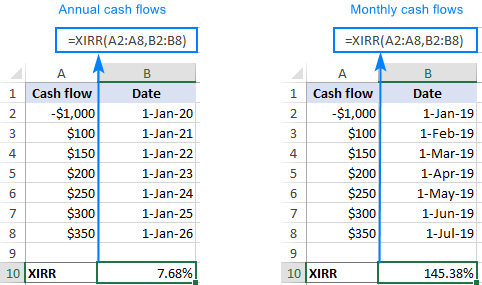To find an approximate monthly XIRR, you can use the below calculation, where E1 is the result of the regular XIRR formula:

`=(1+E1)^(1/12)-1`

Or you can embed XIRR directly in the equation:

`=(1+XIRR(A2:A8,B2:B8))^(1/12)-1`

As an additional check, let's use the IRR function on the same cash flows. Please keep in mind that IRR will also compute an approximate rate because it assumes all the time periods to be equal:

`=IRR(A2:A8)`

As the result of these calculations, we get a monthly XIRR of 7.77%, which is very close to 7.68% produced by the IRR formula: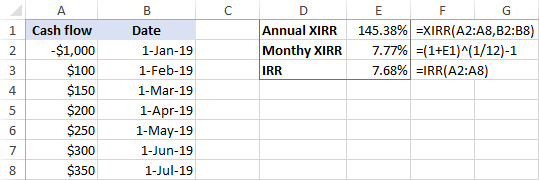The conclusion: if you are looking for an annualized IRR for monthly cash flows, use the XIRR function in its pure form; to get a monthly IRR, apply the adjustment described above.

## Excel XIRR template

To quickly get the internal rate of return for different projects, you can create a versatile XIRR calculator for Excel. Here's how:

1. Input the cash flows and dates in two individual columns (A and B in this example).
2. Create two dynamic defined ranges, named Cash_flows and Dates. Technically, that will be named formulas:

Cash_flows:

`=OFFSET(Sheet1!\$A\$2,0,0,COUNT(Sheet1!\$A:\$A),1)`

Dates:

`=OFFSET(Sheet1!\$B\$2,0,0,COUNT(Sheet1!\$B:\$B),1)`

Where Sheet1 is the name of your worksheet, A2 is the first cash flow, and B2 is the first date.

For the detailed step-by-step instructions, please see How to create a dynamic named range in Excel.

3. Supply the dynamic defined names that you've created to the XIRR formula:

`=XIRR(Cash_flows, Dates)`

Done! You can now add or remove as many cash flows as you want, and your dynamic XIRR formula will recalculate accordingly: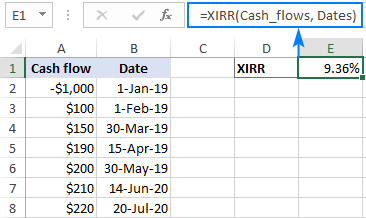## XIRR vs. IRR in Excel

The main difference between Excel XIRR and IRR functions is this:

• IRR assumes that all the periods in a series of cash flows are equal. You use this function to find the internal rate of return for periodic cash flows such as monthly, quarterly or annual.
• XIRR allows you to assign a date to each individual cash flow. So, use this function to calculate IRR for cash flows that are not necessarily periodic.

Generally, if you know the exact dates of the payments, it is advisable to use XIRR because it provides better calculation accuracy.

As an example, let's compare the results of IRR and XIRR for the same cash flows:

If all the payments occur at regular intervals, the functions return very close results: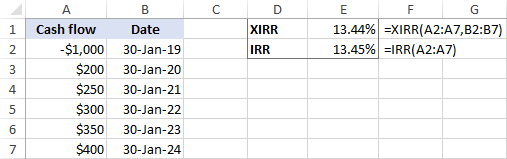If the timing of cash flows is unequal, the difference between the results is quite significant: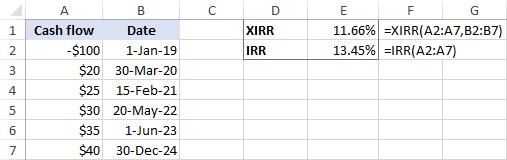## XIRR and XNPV in Excel

XIRR is closely related to the XNPV function because the result of XIRR is the discount rate that leads to a zero net present value. In other words, XIRR is XNPV = 0. The following example demonstrates the relationship between XIRR and XNPV in Excel.

Let's say you are considering some investment opportunity and want to examine both the net present value and the internal rate of return on this investment.

With the cashflows in A2:A5, dates in B2:B5 and the discount rate in E1, the following XNPV formula will give you the net present value of the future cash flows:

`=XNPV(E1, A2:A5, B2:B5)`

A positive NPV indicates that the project is profitable: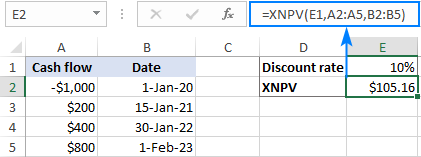Now, let's find what discount rate will make the net present value zero. For this, we use the XIRR function:

`=XIRR(A2:A5, B2:B5)`

To check if the rate produced by XIRR really leads to a zero NPV, put it in the rate argument of your XNPV formula:

`=XNPV(E4, A2:A5, B2:B5)`

Or embed the entire XIRR function:

`=XNPV(XIRR(A2:A5, B2:B5), A2:A5, B2:B5)`

Yep, the XNPV rounded to 2 decimal places does equal zero: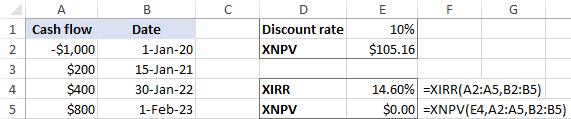To display the exact NPV value, choose to show more decimal places or apply the Scientific format to the XNPV cell. That will produce a result similar to this: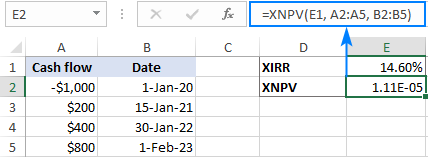If you are not familiar with the scientific notation, perform the following calculation to convert it to a decimal number:

`1.11E-05 = 1.11*10^-5 = 0.0000111`

## Excel XIRR function not working

If you have run into a problem with the XNPV function in Excel, below are the main points to check.

### #NUM! error

A #NUM error may occur because of the following reasons:

• The values and dates ranges have different lengths (different number of columns or rows).
• The values array does not contain at least one positive and one negative value.
• Any of the subsequent dates are earlier than the first date.
• A result is not found after 100 iterations. In this case, try a different guess.

For more details, see How to fix #NUM error in Excel.

### #VALUE! error

A #VALUE error may be caused by the following:

• Any of the supplied values are non-numeric.
• Some of the supplied dates cannot be identified as valid Excel dates.

That's how you calculate XIRR in Excel. To have a closer look at the formulas discussed in this tutorial, you are welcome to download our sample workbook below. I thank you for reading and hope to see you on our blog next week!

XIRR Excel template (.xlsx file)